# Parallel Circular Loops Magnetic Flux

A circular loop of radius 0.3 cm lies parallel to a much bigger circular loop of radius 20 cm. The centre of the small loop is on the axis of the bigger loop. The distance between their centres is 15 cm. If a current of 2.0 A flows through the smaller loop, then the flux linked with bigger loop is:

(1) 9.1 x 10-11 weber    (2) 6 x 10-11 weber    (3) 3.3 x 10-11 weber    (4) 6.6 x 10-9 weber

[JEE Main 2013]

# $xMnO_4^ - + y{C_2}O_4^{2 - } + z{H^ + } \to xM{n^{2 + }} + 2yC{O_2} + \frac{z}{2}{H_2}O$

Consider the following reaction: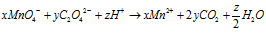The values of x, y and z in the reaction are, respectively:

(1) 5, 2 and 16        (2) 2, 5 and 8        (3) 2, 5 and 16        (4) 5, 2 and 8

[JEE Main 2013]

# Vectors $\overrightarrow {AB} = 3\hat i + 4\hat k$ and $\overrightarrow {AC} = 5\hat i - 2\hat j + 4\hat k$

If the vectors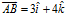and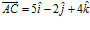are the sides of a triangle ABC, then the length of the median through A is:

(1)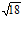(2)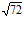(3)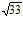(4)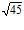[JEE Main 2013]

# A projectile is given an initial velocity of $\hat i + 2\hat j$

A projectile is given an initial velocity of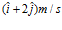, whereis along the ground and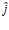is along the vertical. If g = 10 m/s2, the equation of its trajectory is:

(1)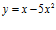(2)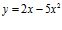(3)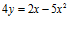(4)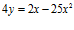[JEE Main 2013]

# Plano-Convex Lens Having 3 mm Thickness

Diameter of a plano – convex lens is 6 cm and thickness at the centre is 3 mm. If speed of light in material of lens is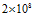m/s, the focal length of the lens is:

(1) 15 cm    (2) 20 cm    (3) 30 cm    (4) 10 cm

[JEE Main 2013]

Continue reading Plano-Convex Lens Having 3 mm Thickness